Anúncio

# lecture 2.5.pptx

1 de Feb de 2023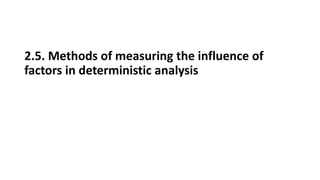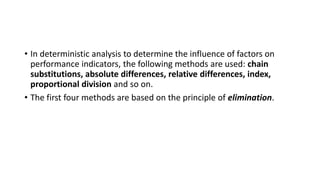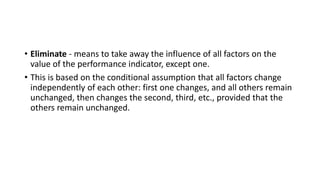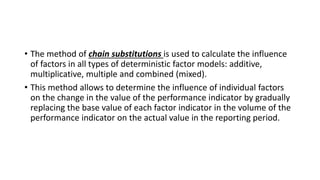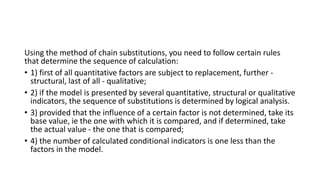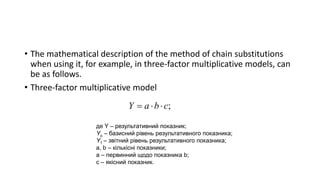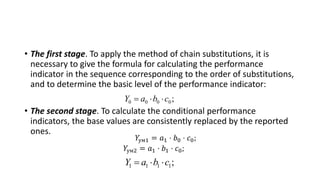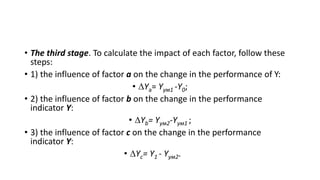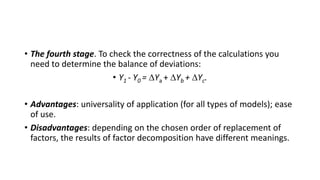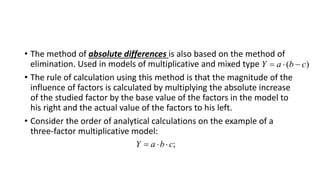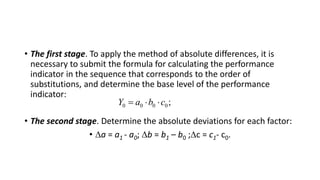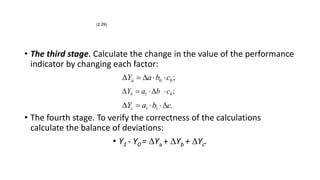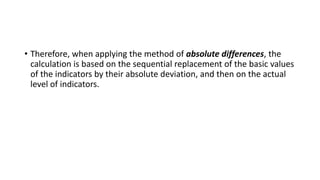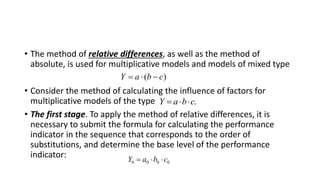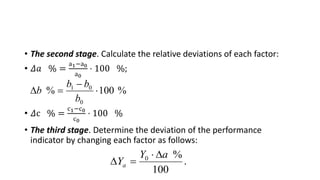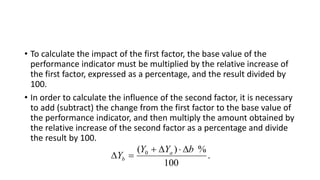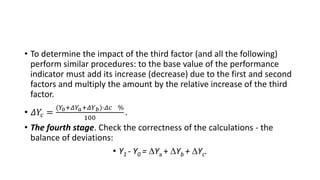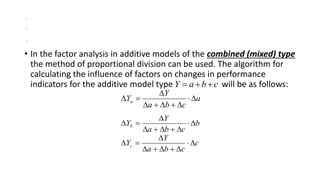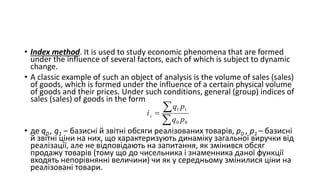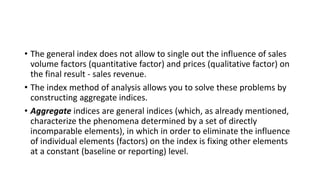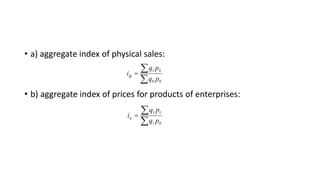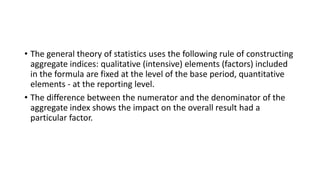1 de 22
Anúncio

### lecture 2.5.pptx

1. 2.5. Methods of measuring the influence of factors in deterministic analysis
2. • In deterministic analysis to determine the influence of factors on performance indicators, the following methods are used: chain substitutions, absolute differences, relative differences, index, proportional division and so on. • The first four methods are based on the principle of elimination.
3. • Eliminate - means to take away the influence of all factors on the value of the performance indicator, except one. • This is based on the conditional assumption that all factors change independently of each other: first one changes, and all others remain unchanged, then changes the second, third, etc., provided that the others remain unchanged.
4. • The method of chain substitutions is used to calculate the influence of factors in all types of deterministic factor models: additive, multiplicative, multiple and combined (mixed). • This method allows to determine the influence of individual factors on the change in the value of the performance indicator by gradually replacing the base value of each factor indicator in the volume of the performance indicator on the actual value in the reporting period.
5. Using the method of chain substitutions, you need to follow certain rules that determine the sequence of calculation: • 1) first of all quantitative factors are subject to replacement, further - structural, last of all - qualitative; • 2) if the model is presented by several quantitative, structural or qualitative indicators, the sequence of substitutions is determined by logical analysis. • 3) provided that the influence of a certain factor is not determined, take its base value, ie the one with which it is compared, and if determined, take the actual value - the one that is compared; • 4) the number of calculated conditional indicators is one less than the factors in the model.
6. • The mathematical description of the method of chain substitutions when using it, for example, in three-factor multiplicative models, can be as follows. • Three-factor multiplicative model ; c b a Y    де Y – результативний показник; Yо – базисний рівень результативного показника; Y1 – звітний рівень результативного показника; а, b – кількісні показники; а – первинний щодо показника b; с – якісний показник.
7. • The first stage. To apply the method of chain substitutions, it is necessary to give the formula for calculating the performance indicator in the sequence corresponding to the order of substitutions, and to determine the basic level of the performance indicator: • The second stage. To calculate the conditional performance indicators, the base values are consistently replaced by the reported ones. ; 0 0 0 0 c b a Y    𝑌ум1 = 𝑎1 ⋅ 𝑏0 ⋅ 𝑐0; 𝑌ум2 = 𝑎1 ⋅ 𝑏1 ⋅ 𝑐0; ; 1 1 1 1 c b a Y   
8. • The third stage. To calculate the impact of each factor, follow these steps: • 1) the influence of factor a on the change in the performance of Y: • Yа= Yум1 -Y0; • 2) the influence of factor b on the change in the performance indicator Y: • Yb= Yум2-Yум1 ; • 3) the influence of factor c on the change in the performance indicator Y: • Yс= Y1 - Yум2.
9. • The fourth stage. To check the correctness of the calculations you need to determine the balance of deviations: • Y1 - Y0 = Yа + Yb + Yс. • Advantages: universality of application (for all types of models); ease of use. • Disadvantages: depending on the chosen order of replacement of factors, the results of factor decomposition have different meanings.
10. • The method of absolute differences is also based on the method of elimination. Used in models of multiplicative and mixed type • The rule of calculation using this method is that the magnitude of the influence of factors is calculated by multiplying the absolute increase of the studied factor by the base value of the factors in the model to his right and the actual value of the factors to his left. • Consider the order of analytical calculations on the example of a three-factor multiplicative model: ) ( c b a Y    ; c b a Y   
11. • The first stage. To apply the method of absolute differences, it is necessary to submit the formula for calculating the performance indicator in the sequence that corresponds to the order of substitutions, and determine the base level of the performance indicator: • The second stage. Determine the absolute deviations for each factor: • а = а1 - а0; b = b1 – b0 ;c = с1- с0. ; 0 0 0 0 c b a Y   
12. • The third stage. Calculate the change in the value of the performance indicator by changing each factor: • The fourth stage. To verify the correctness of the calculations calculate the balance of deviations: • Y1 - Y0 = Yа + Yb + Yс. ; 0 0 c b a Yà      ; 0 1 c b a Yb      . 1 1 c b a Yc      (2.29)
13. • Therefore, when applying the method of absolute differences, the calculation is based on the sequential replacement of the basic values of the indicators by their absolute deviation, and then on the actual level of indicators.
14. • The method of relative differences, as well as the method of absolute, is used for multiplicative models and models of mixed type • Consider the method of calculating the influence of factors for multiplicative models of the type • The first stage. To apply the method of relative differences, it is necessary to submit the formula for calculating the performance indicator in the sequence that corresponds to the order of substitutions, and determine the base level of the performance indicator: ) ( c b a Y    . c b a Y    0 0 0 0 c b a Y   
15. • The second stage. Calculate the relative deviations of each factor: • 𝛥𝑎 % = а1−а0 а0 ⋅ 100 %; • 𝛥с % = с1−с0 с0 ⋅ 100 % • The third stage. Determine the deviation of the performance indicator by changing each factor as follows: % 100 % 0 0 1     b b b b . 100 % 0 a Y Ya    
16. • To calculate the impact of the first factor, the base value of the performance indicator must be multiplied by the relative increase of the first factor, expressed as a percentage, and the result divided by 100. • In order to calculate the influence of the second factor, it is necessary to add (subtract) the change from the first factor to the base value of the performance indicator, and then multiply the amount obtained by the relative increase of the second factor as a percentage and divide the result by 100. . 100 % ) ( 0 b Y Y Y a b      
17. • To determine the impact of the third factor (and all the following) perform similar procedures: to the base value of the performance indicator must add its increase (decrease) due to the first and second factors and multiply the amount by the relative increase of the third factor. • 𝛥𝑌с = (𝑌0+𝛥𝑌𝑎+𝛥𝑌𝑏)⋅𝛥𝑐 % 100 . • The fourth stage. Check the correctness of the calculations - the balance of deviations: • Y1 - Y0 = Yа + Yb + Yс.
18. • In the factor analysis in additive models of the combined (mixed) type the method of proportional division can be used. The algorithm for calculating the influence of factors on changes in performance indicators for the additive model type will be as follows: c b a Y    a c b a Y Ya           b c b a Y Yb           c c b a Y Yc           ; ; .
19. • Index method. It is used to study economic phenomena that are formed under the influence of several factors, each of which is subject to dynamic change. • A classic example of such an object of analysis is the volume of sales (sales) of goods, which is formed under the influence of a certain physical volume of goods and their prices. Under such conditions, general (group) indices of sales (sales) of goods in the form • де q0 , q1 – базисні й звітні обсяги реалізованих товарів, p0 , p1 – базисні й звітні ціни на них, що характеризують динаміку загальної виручки від реалізації, але не відповідають на запитання, як змінився обсяг продажу товарів (тому що до чисельника і знаменника даної функції входять непорівнянні величини) чи як у середньому змінилися ціни на реалізовані товари.    0 0 1 1 p q p q iз
20. • The general index does not allow to single out the influence of sales volume factors (quantitative factor) and prices (qualitative factor) on the final result - sales revenue. • The index method of analysis allows you to solve these problems by constructing aggregate indices. • Aggregate indices are general indices (which, as already mentioned, characterize the phenomena determined by a set of directly incomparable elements), in which in order to eliminate the influence of individual elements (factors) on the index is fixing other elements at a constant (baseline or reporting) level.
21. • a) aggregate index of physical sales: • b) aggregate index of prices for products of enterprises:    0 0 0 1 p q p q iф    0 1 1 1 p q p q iц
22. • The general theory of statistics uses the following rule of constructing aggregate indices: qualitative (intensive) elements (factors) included in the formula are fixed at the level of the base period, quantitative elements - at the reporting level. • The difference between the numerator and the denominator of the aggregate index shows the impact on the overall result had a particular factor.
Anúncio